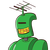# In an AP the sum ofsecond & third term is 22 & theProduct of first & fourth term is85. Then find th

In an AP the sum of
second & third term is 22 & the
Product of first & fourth term is
85. Then find the sum of first
10 terms by considering the
positive value of common
difference.​

### 1 thought on “In an AP the sum of<br />second & third term is 22 & the<br />Product of first & fourth term is<br />85. Then find th”

1.When the consecutive terms of series differ by a common number, then the series is said to be Arithmetic Progression

Let a be the first term of the AP

d be the common difference of the AP

nth term of AP ⇒ a + ( n – 1) d

Given,

The sum of second and third term is 22

⇒ (a + d) + (a + 2d) =22

⇒ 2a + 3d = 22

⇒ d = 1/3 ( 22 – 2a)

The product of first and fourth term is 85

⇒ a ( a + 3d) = 85

⇒ a² + 3ad = 85

Substituting the value of d gives,

⇒ a ( a + 3 ( 1/3 * (22 – 2a) )) = 85

⇒ a ( a + 22 – 2a) = 85

⇒ a ( – a + 22) = 85

⇒ – a² + 22a = 85

⇒ a² – 22a + 85 = 0

⇒ a² – 17a – 5a + 85 = 0

⇒ a ( a – 17) – 5 ( a – 17)= 0

⇒ (a-5)(a-17)= 0

⇒ a = 5 or a = 17

If a = 5,

d = 1/3 ( 22 – 10) = 1/3 ( 12) = 4

If a = 17,

d = 1/3 ( 22 – 34) = 1/3 ( – 12) = – 4

a = 5, d = 4

Then Arithmetic Progression is

5, 9, 13, 17

a = 17, d = – 4

Then Arithmetic Progression is,

17, 13, 9, 5

Therefore, The required terms in the AP are 5, 9, 13, 17.